## Exoteric or esoteric?

### Multivariate analysis

There are days when I feel biblical. Other days I feel mythological. Today I feel philosophical and even a little Masonic.

And the reason is that the other day it gave me to wonder what the difference between exoteric and esoteric were, so I consulted with that friend of us all who knows so much about everything, our friend Google. It kindly explained to me that both terms are similar and usually explain two aspects of the same doctrine. Exoterism refers to that knowledge that is not limited to a certain group in the community that deals with that doctrine, and that can be disclosed and made available to anyone. On the other hand, esotericism refers to knowledge that belongs to a deeper and higher order, only available to a privileged few specially educated to understand it.

And now, once the difference is understood, I ask you a slightly tricky question: is multivariate statistics exoteric or esoteric? The answer, of course, will depend on each one, but we are going to see if it is true that both concepts are not contradictory, but complementary, and we can strike a balance between both of them, at least in understanding the usefulness of multivariate techniques.

#### Multivariate analysis

We are more used to work with univariate or bivariate statistical techniques, which allow us to study together a maximum of two characteristics of the individuals in a population to detect relationships between them.

However, with the mathematical development and, above all, the calculating power of modern computers, multivariate statistical techniques are becoming increasingly important.

We can define multivariate analysis as the set of statistical procedures that simultaneously study various characteristics of the same subject or entity, in order to analyze the interrelation that may exist among all the random variables that these characteristics represent. Let me insist on the two aspects of these techniques: the multiplicity of variables and the study of their possible interrelations.

There are many multivariate analysis techniques, ranging from purely descriptive methods to those that use statistical inference techniques to draw conclusions from the data and that are able to develop models that are not obvious to the naked eye by observing the data obtained. They will also allow us to develop prediction models of various variables and establish relationships among them.

Some of these techniques are the extension of their equivalents with two variables, one dependent and the other independent or explanatory. Others have nothing similar in two-dimensional statistics.

Some authors classify these techniques into three broad groups: full-range and non-full-range models, techniques to reduce dimensionality, and classification and discrimination methods. Do not worry if this seems gibberish, we will try to simplify it a bit.

To be able to talk about the FULL AND NON-FULL RANGE TECHNIQUES, I think it will be necessary to explain first what range we are talking about.

#### A previous digress

Although we are not going to go into the subject in depth, all these methods involve matrix calculation techniques within them. You know, matrices or arrays, a set of two-dimensional numbers (the ones we are going to discuss here) that form rows and columns and that can be added and multiplied together, in addition to other calculations.

The range of an array is defined as the number of rows or columns that are linearly independent (no matter rows or columns, the number is the same). The range can value from 0 to the minimum number of rows or columns. For example, a 2 row by 3 column array may have a range from 0 to 2. A 5 row by 3 column array may have a range from 0 to 3. Now imagine an array with two rows, the first 1 2 3 and the second 3 6 9 (it has 3 columns). Its maximum range would be 2 (the smallest number of rows and columns) but, if you look closely, the second row is the first one multiplied by 3, so there is only one linearly independent row, so its range is equal to 1.

Well, an array is said to be a full-range one when its range is equal to the largest possible for an array of the same dimensions. The third example that I have given you would be a non-full range array, since a 2×3 matrix would have a maximum range of 2 and that of our array is 1.

Once this is understood, we go with the full and non-full range methods.

#### Multiple linear regression

The first one we will look at is the multiple linear regression model. This model, an extension of the simple linear regression model, is used when we have a dependent variable and a series of explanatory variables, all of them quantitative variables, and they can be linearly related and the explanatory variables form a full-range array.

Like simple regression, this technique allows us to predict changes in the dependent variable based on the values of explanatory variables. The formula is like that of the simple regression, but including all the explanatory independent variables, so I’m not going to bore you with it. However, since I have punished you with ranges and matrices, let me tell you that, in arrays terms, it can be expressed as follows:

Y = Xβ + ei

where X is the full range matrix of the explanatory variables. The equation includes an error term that is justified by the possible omission in the model of relevant explanatory variables or measurement errors.

#### Canonical correlation

To complicate matters, imagine that we were to simultaneously correlate several independent variables with several dependent ones. In this case, multiple regression does not help us, and we would have to resort to the canonical correlation technique, which allows us to make predictions of various dependent variables based on the values of several explanatory variables.

#### Non-full range techniques

If you remember bivariate statistics, analysis of variance (ANOVA) is the technique that allows us to study the effect on a quantitative dependent variable of explanatory variables when these are categories of a qualitative variable (we call these categories as factors). In this case, since each observation can belong to one and only one of the factors of the explanatory variable, matrix X will be of a non-full range one.

A slightly more complicated situation occurs when the explanatory variables are a quantitative variable and one or more factors of a qualitative variable. On these occasions we resorted to a generalized linear model called the analysis of covariance (ANCOVA).

Transferring what we have just said to the realm of multivariate statistics, we would have to use the extension of these techniques. The extension of ANOVA when there is more than one dependent variable that cannot be combined into one is the multivariate analysis of variance (MANOVA). If factors of qualitative variables coexist with quantitative variables, we will resort to the multivariate analysis of covariance (MANCOVA).

The second group of multivariate techniques are those that try the REDUCTION OF DIMENSIONALITY.

Sometimes we must handle such a high number of variables that it is complex to organize them and reach some useful conclusion. Now, if we are lucky that the variables are correlated with each other, the information provided by the set will be redundant, since the information given by some variables will include that already provided by other variables in the set.

In these cases, it is useful to reduce the dimension of the problem by decreasing the number of variables to a smaller set of variables that are not correlated with each other and that collect most of the information included in the original set. And we say most of the information because, obviously, the more we reduce the number, the more information we will lose.

The two fundamental techniques that we will use in these cases are principal component analysis and factor analysis.

#### Principal component analysis

Principal component analysis takes a set of p correlated variables and transforms them into a new set of uncorrelated variables, which we call principal components. These main components allow us to explain the variables in terms of their common dimensions.

Without going into detail, a correlation matrix and a series of vectors are calculated that will provide us with the new main components, ordered from highest to lowest according to the variance of the original data that each component encompass. Each component will be a linear combination of the original variables, somewhat like a regression line.

Let’s imagine a very simple case with six explanatory variables (X1 to X6). Principal component 1 (PC1) can be, let’s say, 0.15X1 + 0.5X2 – 0.6X3 + 0.25X4 – 0.1X5 – 0.2X6 and, in addition, encompass 47% of the total variance. If PC2 turns out to encompass 30% of the variance, with PC1 and PC2 we will have 77% of the total variance controlled with a data set that is easier to handle (let’s think if instead of 6 variables we have 50). And not only that, if we represent graphically PC1 versus PC2, we can see if some type of grouping of the variable under study occurs according to the values of the principal components.

In this way, if we are lucky and a few components collect most of the variance of the original variables, we will have reduced the dimension of the problem. And although, sometimes, this is not possible, it can always help us to find groupings in the data defined by a large number of variables, which links us to the following technique, factor analysis.

#### Factor analysis

We know that the total variance of our data (the one studied by principal component analysis) is the sum of three components: the common or shared variance, the specific variance of each variable, and the variance due to chance and measurement errors. Again, without going into detail, the factor analysis method starts from the correlation matrix to isolate only the common variance and try to find a series of common underlying dimensions, called factors, that are not observable by looking at the original set of variables.

As we can see, these two methods are very similar, so there is a lot of confusion about when to use one and when another, especially considering that principal component analysis may be the first step in the factor analysis methodology.

As we have already said, principal component analysis tries to explain the maximum possible proportion of the total variance of the original data, while the objective of the factor analysis study is to explain the covariance or correlation that exists among its variables. Therefore, principal component analysis will usually be used to search for linear combinations of the original variables and reduce one large data set to a smaller and more manageable one, while we will resort to factor analysis when looking for a new set of variables, generally smaller than the original, and to represent what the original variables have in common.

Moving forward on our arduous today’s path, for those hard-working who are still reading, we are going to discuss CLASSIFICATION AND DISCRIMINATION METHODS, which are two: cluster analysis and discriminant analysis.

#### Cluster analysis

Cluster analysis tries to recognize patterns to summarize the information contained in the initial set of variables, which are grouped according to their greater or less homogeneity. In summary, we look for groups that are mutually exclusive, so that the elements are as similar as possible to those of their group and as different as possible to those of the other groups.

The most famous part of the cluster analysis is, without a doubt, its graphic representation, with decision trees and dendrograms, in which homogeneous groups increasingly different from those farthest between the branches of the tree are represented.

But, instead of wanting to segment the population, let’s assume that we already have a population segmented into a number of classes, k. Suppose we have a group of individuals defined by a number p of random variables. If we want to know to what class of the population a certain individual may belong, we will resort to the technique of discriminant analysis.

#### Discriminant analysis

Suppose that we have a new treatment that is awfully expensive, so we only want to give it to patients who we are sure that they will comply with the treatment. Thus, our population is segmented into compliant and non-compliant classes. It would be very useful for us to select a set of variables that would allow us to discriminate which class a specific person can belong to, and even which of these variables are the ones that best discriminate between the two groups. Thus, we will measure the variables in the candidate for treatment and, using what is known as a discrimination criterion or rule, we will assign it to one or the other group and proceed accordingly. Of course, do not forget, there will always be a probability of being wrong, so we will be interested in finding the discriminant rule that minimizes the probability of discrimination error.

Discriminant analysis may seem similar to cluster analysis, but if we think about it, the difference is clear. In the discriminant analysis the groups are previously defined (compliant or non-compliant, in our example), while in the cluster analysis we look for groups that are not evident: we would analyze the data and discover that there are patients who do not take the pill that we give them, something that had not even crossed our minds (in addition to our ignorance, we would demonstrate our innocence).

#### We are leaving…

And here we are going to leave it for today. We have flown over the steep landscape of multivariate statistics from a great height and I hope it has served to transfer it from the field of the esoteric to that of the exoteric (or was it the other way around?). We have not entered the specific methodology of each technique, since we could have written an entire book. By roughly understanding what each method is and what it is for, I think we have quite a lot. In addition, statistical packages carried them out, as always, effortlessly.

Also don’t you think that we have talked about all the methods that have been developed for multivariate analysis. There are many others, such as conjoint analysis and multidimensional scaling, widely used in advertising to determine the attributes of an object that are preferred by the population and how they influence their perception of it. We could also get lost among other newer techniques, such as correspondence analysis, or linear probability models, such as logit and probit analysis, which are combinations of multiple regression and discriminant analysis, not to mention simultaneous or structural equation models. But that is another story…

## By your actions they will judge you

### Sample size calculation in survival studies

Today you are going to forgive me, but I am in a mood a little biblical. And I was thinking about the sample size calculation for survival studies and it reminded me of the message that Ezekiel transmits to us: according to your ways and your works they will judge you.

Once again, you will think that from all the buzzing of evidence-based medicine in my head I have gone a little nuts, but if you hold on a bit and continue reading, you will see that the analogy can be explained.

#### A little introduction

One of the most valued methodological quality indicators of a study is the previous calculation of the sample size necessary to demonstrate (or reject) the working hypothesis. When we want to study the effect of an intervention, we must, a priori, define what effect size we want to detect and calculate the sample size necessary to be able to do it, as long as the effect exists (something we want when we plan the experiment, but which we do not know a priori) , taking into account the level of significance and the power that we want the study to have .

In summary, if we detect the effect size that we previously established, the difference between the two groups will be statistically significant (our desired p <0.05). On the contrary, if there is no significant difference, there is probably no real difference, although always with the risk of making a type 2 error that is equal to 1 minus the power of the study.

So far it seems clear, we must calculate the number of participants we need. But this is not so simple for survival studies.

#### The approach to the problem

Survival studies grouped a series of statistical techniques to deal with situations in which it is not enough to observe an event, it is critical the time that elapses until the event occurs. In these cases, the outcome variable will be neither quantitative nor qualitative, but from time to event. It is a mixed variable type that would have a dichotomous part (the event occurs or does not) and a quantitative part (how long it takes to occur).

The name of survival studies is a bit misleading and one can think that the event under study will be the death of the participants, but nothing is further from reality. The event can be any type of incident or occurrence, good or bad for the participant. What happens is that the first studies were applied to situations in which the event of interest was death and the name has prevailed.

In these studies, the participants’ follow-up period is often uneven, and some may even end the study without reporting the event of interest or missing out of the study before it ends.

For these reasons, if we want to know if there are differences between the presentation of the event of interest in the two branches of the study, the number of subjects participating will not be so important to calculate the sample, but rather the number of events that we need for the difference to be significant if the clinically important difference is reached, which we must establish a priori.

Let’s see how it is done, depending on the type of contrast we plan to use.

#### Sample size calculation in survival studies

If we only want to determine the number of necessary events that we have to observe to detect a difference between a certain group and the population from which it is sourced, the formula to do so is as follows: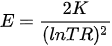Where E is the number of events we need to observe, K is the value determined by the confidence level and the power of the study and lnRR is the natural logarithm of the risk rate.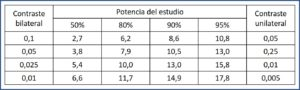The value of K is calculated as (Zα + Zβ)2, with z being the standardized value for the chosen confidence and power level. The most common is to perform a bilateral contrast (with two tails) with a confidence level of 0.05 and a power of 80%. In this case, the values ​​are Zα = 1.96, Zβ = 0.84 and K = 7.9. In the attached table I leave you the most frequent values ​​of K, so you do not have to calculate them.

The risk rate is the ratio between the risk of the study group and the risk in the population, which we are supposed to know. It is defined as Sm1 /Sm2, where Sm1 is the mean time of appearance of the event in the population and Sm2 is the expected in the study group.

Let’s give an example to better understand what has been said so far.

Suppose that patients or treatment with a certain drug (which we will call A to not work ridiculously hard) are at risk of developing a stomach ulcer during the first year of treatment. Now we select a group and give them a treatment (B, this time) that acts as prophylaxis, in such a way that we hope that the event will take another year to occur. How many ulcers do we have to observe in a study with a confidence level of 0.05 and a power of 0.8 (80%)?

We know that K is worth 7.9. Sm1 = 1 and Sm2 = 2. We substitute their values ​​in the formula that we already know: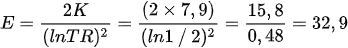We will need to see 33 ulcers during follow-up. Now we can calculate how many patients we must include in the study (I find it difficult to enroll just ulcers).

Let’s assume that we can enroll 12 patients a year. If we want to observe 33 ulcers, the follow-up should last for 33/12 = 2.75, that is, 3 years. For more security, we would plan a slightly higher follow-up.

#### Survival curve comparison

This was the simplest problem. When we want to compare the two survival curves (we plan to do a log-rank test), the calculation of the sample size is a bit more complex, but not much. After all, we will already be comparing the survival probability curves of the two groups.

In these cases, the formula for calculating the number of necessary events is as follows: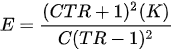We find a new parameter, C, which is the ratio of participants between one group and the other (1: 1, 1: 2, etc.).

But there is another difference with the previous assumption. In these cases, the RR is calculated as the quotient of the natural logarithms of π1 and π2, which are the proportions of participants from each group that present the event in a given period of time.

Following the previous example, suppose we know that the ulcer risk in those who are on A is 50% in the first 6 months and that of those who are on B, 20%. How many ulcers do we need to observe with the same level of confidence and the same power of the study?

Let’s substitute the values ​​in the previous formula: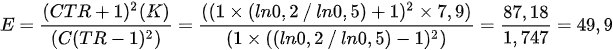We will need to observe 50 ulcers during the study. Now we need to know how many participants (not events) we need in each branch of the study. We can obtain it with the following formula: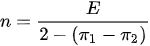If we substitute our values ​​in the equation, we obtain a value of 29.4, so we will need 30 patients in each branch of the study, 60 in all.

Coming to the end, let’s see what would happen if we want a different ratio of participants instead of the easiest, 1: 1. In that case, the calculation of n with the last formula must be adjusted taking into account this proportion, which is our known C: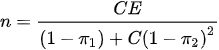Imagine we want a 2:1 ratio. We substitute the values ​​in the equation: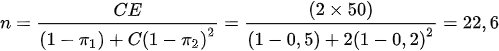We would need 23 participants in one branch and 46, double, in the other, 69 in all.

#### We’re leaving…

And here we leave it for today. As always, everything we have said in this post is so that we can understand the fundamentals of calculating the necessary sample size in this type of study, but I advise you to use a statistical program or a calculator if you ever have to do it. There are many available and some are even totally free.

I hope that you understand now about Ezekiel’s message and that, in these studies, the things we do (or suffer) are more important than how many we do (or suffer). We have seen the simplest way to calculate the sample size of a survival study , although we could have bring unnecessary troubles into our lives and have calculated the sample size based on estimates of risks ratios or hazard ratios. But that is another story…

## The shortest distance

### The least squared method

The other day I was trying to measure the distance between Madrid and New York in Google Earth and I found something unexpected: when I tried to draw a straight line between the two cities, it twisted and formed an arc, and there was no way to avoid it.

I wondered if what said about the straight line being the shortest path between two points would not be true. Of course, right away, I realized where the error was: Euclid was thinking about the distance between two points located in a plane and I was drawing the minimum distance between two points located in a sphere. Obviously, in this case the shortest distance is not marked by a straight line, but an arc, as Google showed me.

And since one thing leads to another, this led me to think about what would happen if instead of two points there were many more. This has to do, as some of you already imagine, with the regression line that is calculated to fit a point cloud. Here, as it is easy to understand, the line cannot pass through all the points without losing its straightness, so the statisticians devised a way to calculate the line that is closest to all points on average. The method they use the most is the one they call the least squares method, whose name suggests something strange and esoteric. However, the reasoning for calculating it is much simpler and, therefore, no less ingenious. Let’s see it.

#### The least squared method

The linear regression model makes it possible, once a linear relation has been established, to make predictions about the value of a variable Y knowing the values of a set of variables X1, X2, … Xn. We call the variable Y as dependent, although it is also known as objective, endogenous, criterion or explained variable. For their part, the X variables are the independent variables, also known as predictors, explanatory, exogenous or regressors.

When there are several independent variables, we are faced with a multiple linear regression model, while when there is only one, we will talk about simple linear regression. To make it easier, we will focus, of course, on the simple regression, although the reasoning also applies to multiple one.

As we have already said, linear regression requires that the relationship between the two variables is linear, so it can be represented by the following equation of a straight line: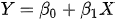Here we find two new friends accompanying our dependent and independent variables: they are the coefficients of the regression model. β0 represents the model constant (also called the intercept) and is the point where the line intersects the ordinate axis (the Y axis, to understand each other better). It would represent the theoretical value of variable Y when variable X values zero.

For its part, β1 represents the slope (inclination) of the regression line. This coefficient tells us the increment of units of variable Y that occurs for each increment of one unit of variable X.

#### We meet chance again

This would be the general theoretical line of the model. The problem is that the distribution of values is never going to fit perfectly to any line, so when we are going to calculate a determined value of Y (yi) from a value of X (xi) there will be a difference between the real value of yi and the one that we obtain with the formula of the line. We have already met with random, our inseparable companion, so we will have no choice but to include it in the equation: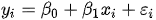Although it seems a similar formula to the previous one, it has undergone a profound transformation. We now have two well-differentiated components, a deterministic and a stochastic (error) component. The deterministic component is marked by the first two elements of the equation, while the stochastic is marked by the error in the estimation. The two components are characterized by their random variable, xi and εi, respectively, while xi would be a specific and known value of the variable X.

Let’s focus a little on the value of εi. We have already said that it represents the difference between the real value of yi in our point cloud and that which would be provided by the equation of the line (the estimated value, represented as ŷi). We can represent it mathematically in the following way: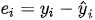This value is known as the residual and its value depends on chance, although if the model is not well specified, other factors may also systematically influence it, but that does not change what we are dealing with.

#### Let’s summarize

Let’s recap what we have so far:

1. A point cloud on which we want to draw the line that best fits the cloud.
2. An infinite number of possible lines, from which we want to select a specific one.
3. A general model with two components: one deterministic and the other stochastic. This second will depend, if the model is correct, on chance.

We already have the values of the variables X and Y in our point cloud for which we want to calculate the line. What will vary in the equation of the line that we select will be the coefficients of the model, β0 and β1. And what coefficients interest us? Logically, those with which the random component of the equation (the error) is as small as possible. In other words, we want the equation with a value of the sum of residuals as low as possible.

Starting from the previous equation of each residual, we can represent the sum of residuals as follows, where n is the number of pairs of values of X and Y that we have: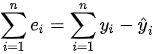But this formula does not work for us. If the difference between the estimated value and the real value is random, sometimes it will be positive and sometimes negative. Furthermore, its average will be zero or close to zero. For this reason, as on other occasions in which it is interesting to measure the magnitude of the deviation, we have to resort to a method that prevents from negatives differences canceling out with the positives ones, so we calculate these squared differences, according to the following formula: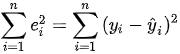At last! We already know where the least squares method comes from: we look for the regression line that gives us the smallest possible value of the sum of the squares of the residuals. To calculate the coefficients of the regression line we will only have to expand the previous equation a little, substituting the estimated value of Y for the terms of the regression line equation: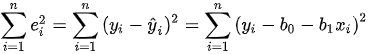and find the values of b0 and b1 that minimize the function. From here the task is a piece of cake, we just have to set the partial derivatives of the previous equation to zero (take it easy, let’s save the hard-mathematical jargon) to get the value of b1: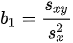Where we have in the numerator the covariance of the two variables and, in the denominator, the variance of the independent variable. From here, the calculation of b0 is a breeze: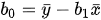We can now build our line that, if you look closely, goes through the mean values of X and Y.

#### A practical exercise

And with this we end the arduous part of this post. Everything we have said is to understand what the least squares mean and where the matter comes from, but it is not necessary to do all this to calculate the linear regression line. Statistical packages do it in the blink of an eye.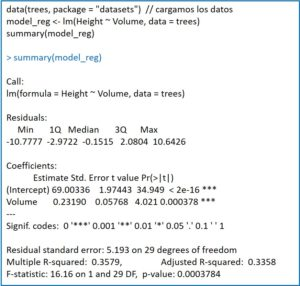For example, in R it is calculated using the function lm(), which stands for linear model. Let’s see an example using the “trees” database (girth, volume and height of 31 observations on trees), calculating the regression line to estimate the volume of the trees knowing their height:

modelo_reg <- lm(Height~Volume, data = trees)

summary(modelo_reg)

The lm() function returns the model to the variable that we have indicated (reg_model, in this case), which we can exploit later, for example, with the summary() function. This will provide us with a series of data, as you can see in the attached figure.

First, the quartiles and the median of the residuals. For the model to be correct, it is important that the median is close to zero and that the absolute values of the residuals are distributed evenly among the quartiles (similar between maximum and minimum and between first and third quartiles).

Next, the point estimate of the coefficients is shown below along with their standard error, which will allow us to calculate their confidence intervals. This is accompanied by the values of the t statistic with its statistical significance. We have not said it, but the coefficients follow a Student’s t distribution with n-2 degrees of freedom, which allows us to know if they are statistically significant.

Finally, the standard deviation of the residuals is provided, the square of the multiple correlation coefficient or determination coefficient (the precision with which the line represents the functional relationship between the two variables; its square root in simple regression is the Pearson’s correlation coefficient), its adjusted value (which will be more reliable when we calculate regression models with small samples) and the F contrast to validate the model (the variance ratios follow a Snedecor’s F distribution).

Thus, our regression equation would be as follows: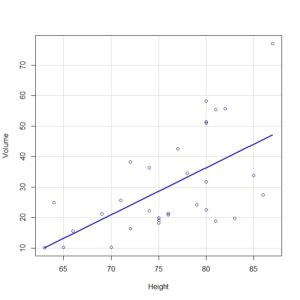Height = 69 + 0.23xVolume

We could already calculate how tall a tree could be given a specific volume that was not in our sample (although it should be within the range of data used to calculate the regression line, since it is risky to make predictions outside this range).

Also, with the scatterplot(Volume ~ Height, regLine = TRUE, smooth = FALSE, boxplots = FALSE, data = trees) command, we could draw the point cloud and the regression line, as you can see in the second figure.

And we could calculate many more parameters related to the regression model calculated by R, but we will leave it here for today.

#### We’re leaving…

Before finishing, just to tell you that the least squares method is not the only one that allows us to calculate the regression line that best fits our point cloud. There is also another method that is that of the maximum likelihood, which gives more importance to the choice of the coefficients that with more compatibility with the observed values. But that is another story…

## Rioja vs Ribera

### Frequentist vs Bayesian statistics

This is one of the typical debates that one can have with a brother-in-law during a family dinner: whether the wine from Ribera is better than that from Rioja, or vice versa. In the end, as always, the brother-in-law will be (or will want to be) right, which will not prevent us from trying to contradict him. Of course, we must make good arguments to avoid falling into the same error, in my humble opinion, in which some fall when participating in another classic debate, this one from the less playful field of epidemiology: Frequentist vs. Bayesian statistics?

And these are the two approaches that we can use when dealing with a research problem.

#### Some previous definitions

Frequentist statistics, the best known and to which we are most accustomed, is the one that is developed according to the classic concepts of probability and hypothesis testing. Thus, it is about reaching a conclusion based on the level of statistical significance and the acceptance or rejection of a working hypothesis, always within the framework of the study being carried out. This methodology forces to stabilize the decision parameters a priori, which avoids subjectivities regarding them.

The other approach to solving problems is that of Bayesian statistics, which is increasingly fashionable and, as its name suggests, is based on the probabilistic concept of Bayes’ theorem. Its differentiating feature is that it incorporates external information into the study that is being carried out, so that the probability of a certain event can be modified by the previous information that we have on the event in question. Thus, the information obtained a priori is used to establish an a posteriori probability that allows us to make the inference and reach a conclusion about the problem we are studying.

This is another difference between the two approaches: while Frequentist statistics avoids subjectivity, Bayesian’s one introduces a subjective (but not capricious) definition of probability, based on the researcher’s conviction, to make judgments about a hypothesis.

Bayesian statistics is not really new. Thomas Bayes’ theory of probability was published in 1763, but experiences a resurgence from the last third of the last century. And as usually happens in these cases where there are two alternatives, supporters and detractors of both methods appear, which are deeply involved in the fight to demonstrate the benefits of their preferred method, sometimes looking more for the weaknesses of the opposite than for their own strengths.

And this is what we are going to talk about in this post, about some arguments that Bayesians use on some occasion that, one more time in my humble opinion, take more advantage misuses of Frequentist statistics by many authors, than of intrinsic defects of this methodology.

#### A bit of history

The history of hypothesis testing begins back in the 20s of the last century, when the great Ronald Fisher proposed to value the working hypothesis (of absence of effect) through a specific observation and the probability of observing a value equal or greater than the observed result. This probability is the p-value, so sacred and so misinterpreted, that it does not mean more than that: the probability of finding a value equal to or more extreme than that found if the working hypothesis were true.

In summary, the p that Fisher proposed is nothing short of a measure of the discrepancy that could exist between the data found and the hypothesis of work proposed, the null hypothesis (H0).

Almost a decade later, the concept of alternative hypothesis (H1) was introduced, which did not exist in Fisher’s original approach, and the reasoning is modified based on two error rates of false positive and negative:

1. Alpha error (type 1 error): probability of rejecting the null hypothesis when, in fact, it is true. It would be the false positive: we believe we detect an effect that, in reality, does not exist.
2. Beta error (type 2 error): it is the probability of accepting the null hypothesis when, in fact, it is false. It is the false negative: we fail to detect an effect that actually exists.

Thus, we set a maximum value for what seems to us the worst case scenario, which is detecting a false effect, and we choose a “small” value. How small is it? Well, by convention, 0.05 (sometimes 0.01). But, I repeat, it is a value chosen by agreement (and there are those who say that it is capricious, because 5% reminds them the fingers of the hand, which are usually 5).

Thus, if p <0.05, we reject H0 in favor of H1. Otherwise, we accept H0, the hypothesis of no effect. It is important to note that we can only reject H0, never demonstrate it in a positive way. We can demonstrate the effect, but not its absence.

Everything said so far seems easy to understand: the frequentist method tries to quantify the level of uncertainty of our estimate to try to draw a conclusion from the results. The problem is that p, which is nothing more than a way to quantify this uncertainty, is sacralized and too often, which is used to their advantage (if I may say so) by opponents of the method to try to expose its weaknesses.

One of the major flaws attributed to the frequentist method is the dependence of the p-value on the sample size. Indeed, the value of p can be the same with a small effect size in a large sample as with a large effect size in a small sample. And this is more important than it may seem at first, since the value that will allow us to reach a conclusion will depend on a decision exogenous to the problem we are examining: the chosen sample size.

Here would be the benefit of the Bayesian method, in which larger samples would serve to provide more and more information about the study phenomenon. But I think this argument is based on a misunderstanding of what an adequate sample is. I am convinced, the more is not always the better.

Another great man, David Sackett, said that “too small samples can be used to prove nothing; samples that are too large can be used to prove nothing ”. The problem is that, in my opinion, a sample is neither large nor small, but sufficient or insufficient to demonstrate the existence (or not) of an effect size that is considered clinically important.

And this is the heart of the matter. When we want to study the effect of an intervention we must, a priori, define what effect size we want to detect and calculate the necessary sample size to be able to do it, as long as the effect exists (something that we desire when we plan the experiment, but that we don’t know a priori) . When we do a clinical trial we are spending time and money, in addition to subjecting participants to potential risk, so it is important to include only those necessary to try to prove the clinically important effect. Including the necessary participants to reach the desired p <0.05, in addition to being uneconomic and unethical, demonstrates a lack of knowledge about the true meaning of p-value and sample size.

This misinterpretation of the p-value is also the reason that many authors who do not reach the desired statistical significance allow themselves to affirm that with a larger sample size they would have achieved it. And they are right, they would have reached the desired p <0.05, but they again ignore the importance of clinical significance versus statistical significance.

When the sample size to detect the clinically important effect is calculated a priori, the power of the study is also calculated, which is the probability of detecting the effect if it actually exists. If the power is greater than 80-90%, the values admitted by convention, it does not seem correct to say that you do not have enough sample. And, of course, if you have not calculated the power of the study before, you should do it before affirming that you have no results due to shortness of sample.

Another argument against the frequentist method and in favor of the Bayesian’s says that hypothesis testing is a dichotomous decision process, in which a hypothesis is rejected or accepted such as you rejects or accepts an invitation to the wedding of a distant cousin you haven’t seen for years.

Well, if they previously forgot about clinical significance, those who affirm this fact forget about our beloved confidence intervals. The results of a study should not be interpreted solely on the basis of the p-value. We must look at the confidence intervals, which inform us of the precision of the result and of the possible values that the observed effect may have and that we cannot further specify due to the effect of chance. As we saw in a previous post, the analysis of the confidence intervals can give us clinically important information, sometimes, although the p is not statistically significant.

#### More arguments

Finally, some detractors of the frequentist method say that the hypothesis test makes decisions without considering information external to the experiment. Again, a misinterpretation of the value of p.

As we already said in a previous post, a value of p <0.05 does not mean that H0 is false, nor that the study is more reliable, or that the result is important (even though the p has six zeros). But, most importantly for what we are discussing now, it is false that the value of p represents the probability that H0 is false (the probability that the effect is real).

Once our results allow us to affirm, with a small margin of error, that the detected effect is real and not random (in other words, when the p is statistically significant), we can calculate the probability that the effect is “real”. And for this, Oh, surprise! we will have to calibrate the value of p with the value of the basal probability of H0, which will be assigned by the researcher based on her knowledge or previous available data (which is still a Bayesian approach).

As you can see, the assessment of the credibility or likelihood of the hypothesis, one of the differentiating characteristics of the Bayesian’s approach, can also be used if we use frequentist methods.

#### We’re leaving…

And here we are going to leave it for today. But before finishing I would like to make a couple of considerations.

First, in Spain we have many great wines throughout our geography, not just Ribera or Rioja. For no one to get offended, I have chosen these two because they are usually the ones asked by the brothers-in-law when they come to have dinner at home.

Second, do not misunderstand me if it may have seemed to you that I am an advocate of frequentist statistics against Bayesian’s. Just as when I go to the supermarket I feel happy to be able to buy wine from various designations of origin, in research methodology I find it very good to have different ways of approaching a problem. If I want to know if my team is going to win a match, it doesn’t seem very practical to repeat the match 200 times to see what average results come out. It  would be better to try to make an inference taking into account the previous results.

And that’s all. We have not gone into depth in what we have commented at the end on the real probability of the effect, somehow mixing both approaches, frequentist’s and Bayesian’s. The easiest way, as we saw in a previous post, is to use a Held’s nomogram. But that is another story…

## A good agreement?

### Kappa interobserver agreement coefficient

We all know that the less we go to the doctor, the best. And this is so for two reasons. First, because if we go to many doctors we are either physically ill or very mentally sick (some unfortunates are both of them). And second, which is the fact I am always struck by, because every doctor tells you something different. And it’s not that doctors don’t know their job, it’s that getting an agreement is not as simple as it seems.

To give you an idea, the problem starts when wanting to know if two doctor who assess the same diagnostic test have a good degree of agreement. Let’s see an example.

#### The director’s problem

Imagine for a moment than I am the manager of a hospital and I want to hire a pathologist because the only one that works at the hospital is overworked.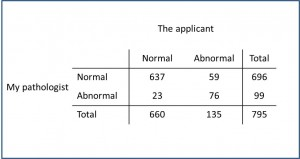I meet with my pathologist and the applicant and give them 795 biopsies to tell me if there’re malignant cells in them. As you can see in the first table, my pathologist finds malignant cells in 99 biopsies, while the applicant sees them in 135 (do not panic, in real life difference couldn’t be so wide, could be?). We wonder what degree of agreement or, rather, concordance exists between the two. The first think that comes to our mind is to calculate the number of biopsies in which they agree: they both agree with 637 normal biopsies and 76 with malignant cells, so the percentages of cases of agreement can be calculated as (637+76)/795=0.896. Hurray!, we think, the two agree almost 90% of the time. The result is not as bad as it seemed to be looking at the table.

But it turns out that when I’m about to hire the new pathologist I wonder if they could have agreed just by chance.

So, a stupid experiment springs to my mind: I take the 795 biopsies and throw a coin, labeling each biopsy as normal if I get heads, or pathological, if tails.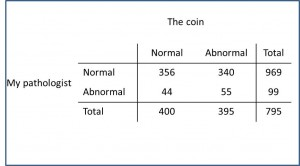The coin says I have 400 normal biopsies and 395 with malignant cells. If I calculate the concordance between the coin and the pathologist, I see that it values (365+55)/795=0.516, 52%!. This is really amazing, just by chance there’s agreement in half of the cases (yes, yes, I know that those know-it-all of you will be thinking that it’s not a surprise, since 50% is the probability of each possible outcome when tossing a coin). So I start thinking how to save money for my hospital and I come out with another experiment that this time is not only stupid, but totally ridiculous: I offer my cousin to do the test instead of throwing a coin (by this time I’m going to left my brother-in-law alone).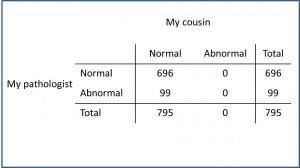The problem, of course, is that my cousin is not a doctor and, although a nice guy, pathology is not his main topic. So, when he starts to see the colorful cells he thinks it’s impossible that such beauty is produced by malignant cells and gives all the biopsies as normal. When we look at the table with the results the first think that we think if to burn it but, for the sake of curiosity, we calculate the concordance between my cousin and my pathologist and see that it’s 696/795=0.875, 87!. Conclusion: it could be more convenient to me to hire my cousin instead of a new pathologist.

At this stage many of you will think that I forgot to take my medication this morning, but the truth is that all these examples serve to show you that, if we want to know what the agreement between two observers is, we must first get rid of the cumbersome and everlasting effect of chance. And for that, mathematicians have invented a statistic called kappa, the interobserver agreement coefficient.

#### The concept of concordance

The function of kappa is to exclude from the observed agreement that part that is due to chance, obtaining a more representative measure of the strength of agreement between observers. Its formula is a ratio in which the numerator is the difference between observed and random difference and which denominator represents the complementary of the random agreement: (Po-Pr) / (1-Pr).

We already know the value of Po with two pathologists: 0.89. To get Pr we have to calculate the theoretical expected values for each cell of the table, in a similar way that we remember we did with chi squared test: the expected value of each cell is the product of the total of its row and column divided by the total of the table. As an example, the expected value of the first cell of our table is (696×660)/795=578. With the expected values we can calculate the probability of agreement due to chance using the same method we used earlier with observed values: (578+17)/795=0.74.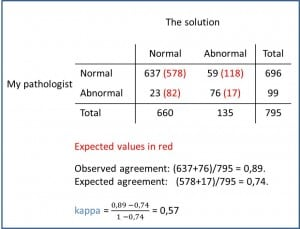And now we can calculate kappa = (0.89-0.74)/(1-0.74) = 0.57. And what can we conclude of a value of 0.57?. We can do with it whatever we want except multiply it by a hundred, because this values doesn’t represent a true percentage. The value of kappa can range between -1 and 1. Negative values indicate that concordance is worse than that expected by chance. A value of 0 indicates that the agreement is similar than that we could get flipping a coin. Values greater than 0 indicate that concordance is slight (0.01-0.20), fair (0.21-0.40), moderate (0.41-0.60), substantial (0.61-0.80) or almost perfect (0.81-1.00). In our case, there’s a fairly good agreement between the two pathologists. If you are curious, you can calculate the kappa for my cousin and you’ll see that it’s no better than flipping a coin.

Kappa can also be calculated if we have measurements of several observers and more than one result for each observation, but tables get so unfriendly that it is better to use a statistical program to calculate it, and by the way, come up with confidence intervals.

Anyway, do not put much trust in kappa, because it needs not to be greater difference among table’s cells. If a cell has few cases the coefficient will tend to underestimate the actual concordance even if it’s very good.

#### We’re leaving…

Finally, say that, although all our examples showed tests with dichotomous result, it’s also possible to calculate interobserver agreement with quantitative results (a rating scale, for instance). Of course, for that we have to use another statistical technique as Bland-Altman’s test, but that’s another story…

## I am Spartacus

I was thinking about the effect size based on mean differences and how to know when that effect is really large and, because of the association of ideas, someone great has come to mind who, sadly, has left us recently. I am referring to Kirk Douglas, that hell of an actor that I will always remember for his roles as a Viking, as Van Gogh or as Spartacus, in the famous scene of the film in which all slaves, in the style of our Spanish’s Fuenteovejuna, stand up and proclaim together to be Spartacus so that Romans cannot do anything to the true one (or to get all equally whacked, much more typical of the modus operandi of the Romans of that time).

You won’t tell me the man wasn’t great. But how great if we compare it with others? How can we measure it? It is clear that not because of the number of Oscars, since that would only serve to measure the prolonged shortsightedness of the so-called academics of the cinema, which took a long time until they awarded him the honorary prize for his entire career. It is not easy to find a parameter that defines the greatness of a character like Issur Danielovitch Demsky, which was the ragman’s son’s name before becoming a legend.

We have it easier to quantify the effect size in our studies, although the truth is that researchers are usually more interested in telling us the statistical significance than in the size of the effect. It is so unusual to calculate it that even many statistical packages forget to have routines to obtain it. In this post, we are going to focus on how to measure the effect size based on differences between means.

Imagine that we want to conduct a trial to compare the effect of a new treatment against placebo and that we are going to measure the result with a quantitative variable X. What we will do is calculate the mean effect between participants in the experimental or intervention group and compare it with the mean of the participants in the control group. Thus, the effect size of the intervention with respect to the placebo will be represented by the magnitude of the difference between the mean in the experimental group and that of the control group:However, although it is the easiest to calculate, this value does not help us to get an idea of the effect size, since its magnitude will depend on several factors, such as the unit of measure of the variable. Let us think about how the differences change if one mean is twice the other as their values are 1 and 2 or 0.001 and 0.002. In order for this difference to be useful, it is necessary to standardize it, so a man named Gene Glass thought he could do it by dividing it by the standard deviation of the control group. He obtained the well-known Glass’ delta, which is calculated according to the following formula:Now, since what we want is to estimate the value of delta in the population, we will have to calculate the standard deviation using n-1 in the denominator instead of n, since we know that this quasi-variance is a better estimator of the population value of the deviation:But do not let yourselves be impressed by delta, it is not more than a Z score (those obtained by subtracting to the value its mean and dividing it by the standard deviation): each unit of the delta value is equivalent to one standard deviation, so it represents the standardized difference in the effect that occurs between the two groups due to the effect of the intervention. This value allows us to estimate the percentage of superiority of the effect by calculating the area under the curve of the standard normal distribution N(0,1) for a specific delta value (equivalent to the standard deviation). For example, we can calculate the area that corresponds to a delta value = 1.3. Nothing is simpler than using a table of values of the standard normal distribution or, even better, the pnorm() function of R, which returns the value 0.90. This means that the effect in the intervention group exceeds the effect in the control group by 90%.

The problem with Glass’ delta is that the difference in means depends on the variability between the two groups, which makes it sensitive to these variance differences. If the variances of the two groups are very different, the delta value may be biased. That is why one Larry Vernon Hedges wanted to contribute with his own letter to this particular alphabet and decided to do the calculation of Glass in a similar way, but using a unified variance that does not assume their equality, according to the following formula:If we substitute the variance of the control group of the Glass’ delta formula with this unified variance we will obtain the so-called Hedges’ g. The advantage of using this unified standard deviation is that it takes into account the variances and sizes of the two groups, so g has less risk of bias than delta when we cannot assume equal variances between the two groups.

However, both delta and g have a positive bias, which means that they tend to overestimate the effect size. To avoid this, Hedges modified the calculation of his parameter in order to obtain an adjusted g, according to the following formula:where df are the degrees of freedom, which are calculated as ne + nc.

This correction is more needed with small samples (few degrees of freedom). It is logical, if we look at the formula, the more degrees of freedom, the less necessary it will be to correct the bias.

So far, we have tried to solve the problem of calculating an estimator of the effect size that is not biased by the lack of equal variances. The point is that, in the rigid and controlled world of clinical trials, it is usual that we can assume the equality of variances between the groups of the two branches of the study. We might think, then, that if this is true, it would not be necessary to resort to the trick of n-1.

Well, Jacob Cohen thought the same, so he devised his own parameter, Cohen’s d. This Cohen’s d is similar to Hedges’ g, but still more sensitive to inequality of variances, so we will only use it when we can assume the equality of variances between the two groups. Its calculation is identical to that of the Hedges’ g, but using n instead of n-1 to obtain the unified variance.

As a rough-and-ready rule, we can say that the effect size is small for d = 0.2, medium for d = 0.5, large for d = 0.8 and very large for d = 1.20. In addition, we can establish a relationship between d and the (r), which is also a widely used measure to estimate the effect size.

The correlation coefficient measures the relationship between an independent binary variable (intervention or control) and a numerical dependent variable (our X). The great advantage of this measure is that it is easier to interpret than the parameters we have seen so far, which all function as standardized Z scores. We already know that r can range from -1 to 1 and the meaning of these values.

Thus, if you want to calculate r given d, you only have to apply the following formula:where p and q are the proportions of subjects in the experimental and control groups (p = ne / n and q = nc / n). In general, the larger the effect size, the greater r and vice versa (although it must be taken into account that r is also smaller as the difference between p and q increases). However, the factor that most determines the value of r is the value of d.

And with this we will end for today. Do not believe that we have discussed all the measures of this family. There are about a hundred parameters to estimate the effect size, such as the determination coefficient, eta-square, chi-square, etc., even others that Cohen himself invented (not very happy with only d), such as f-square or Cohen’s q. But that is another story…

## Columns, sectors, and an illustrious Italian

When you read the title of this post, you can ask yourself with what stupid occurrence am I going to crush the suffered concurrence today, but do not fear, all we are going to do is to put in prospective value that famous aphorism that says that a picture is worth a thousand words. Have I clarified something? I suppose not.

As we all know, descriptive statistics is that branch of statistics that we usually use to obtain a first approximation to the results of our study, once we have finished it.

The first thing we do is to describe the data, for which we make frequency tables and use various measures of tendency and dispersion. The problem with these parameters is that, although they truly represent the essence of the data, it is sometimes difficult to provide a synthetic and comprehensive view with them. It is in these cases that we can resort to another resource, which is none other than the graphic representation of the study results. You know, a picture is worth a thousand words, or so they say.

There are many types of graphs to help us better understand the data, but today we are only going to talk about those that have to do with qualitative or categorical variables.

Remember that qualitative variables represent attributes or categories of the variable. When the variable does not include any sense of order, it is said to be a nominal categorical variable, while if a certain order can be established between the categories, we would say that it is an ordinal categorical variable. For example, the variable “smoker” would be nominal if it has two possibilities: “yes” or “no”. However, if we define it as “occasional”, “little smoker”, “moderate” or “heavy smoker”, there is already a certain hierarchy and we speak of ordinal qualitative variable.

The first type of chart that we are going to consider when representing a qualitative variable is the pie chart. This consists of a circle whose area represents the total data. Thus, an area that will be directly proportional to its frequency is assigned to each category. In this way, the most frequent categories will have larger areas, so that we can get an idea of how the frequencies are distributed in the categories at a glance.

There are three ways to calculate the area of each sector. The simplest is to multiply the relative frequency of each category by 360 °, obtaining the degrees of that sector.

The second is to use the absolute frequency of the category, according to the following rule of three:

Absolute frequency / Total data frequency = Degrees of the sector / 360 °

Finally, the third way is to use the proportions or percentages of the categories:

% of the category / 100% = Degrees of the sector / 360 °

The formulas are very simple, but, in any case, there will be no need to resort to them because the program with which we make the graph will do it for us. The instruction in R is pie(), as you can see in the first figure, in which I show you a distribution of children with exanthematic diseases and how the pie chart would be represented.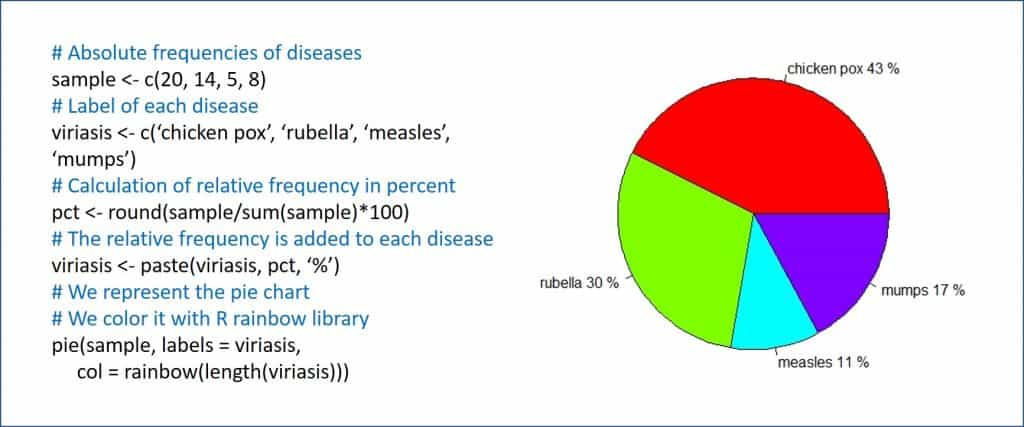The pie chart is designed to represent nominal categorical variables, although it is not uncommon to see pies representing variables of other types. However, and in my humble opinion, this is not entirely correct.

For example, if we make a pie chart for an ordinal qualitative variable, we will be losing information about the hierarchy of the variables, so it would be more correct to use a chart that allows to sort the categories from less to more. And this chart is none other than the bar chart, which we’ll talk about next.

The pie chart will be especially useful when there are few categories of the variable. If there are many, the interpretation is no longer so intuitive, although we can always complete the graph with a frequency table that helps us to better interpret the data. Another tip is to be very careful with 3D effects when drawing cakes. If we go from elaborate, the graphic will lose clarity and will be more difficult to read.

The second graph that we are going to see is, as we have already mentioned, the bar chart, the optimum to represent ordinal qualitative variables. On the horizontal axis, the different categories are represented, and on it some columns or bars are raised whose height is proportional to the frequency of each category. We could also use this type of graph to represent discrete quantitative variables, but what is not very correct to do is use it for the qualitative nominal variables.

The bar chart is able to express the magnitude of the differences between the categories of the variable, but it is precisely its weak point, since it is easily manipulated if we modify the axes’ scales. That is why we must be careful when analyzing this type of graphics to avoid being deceived by the message that the author of the study may want to convey.

This chart is also easy to do with most statistical programs and spreadsheets. The function in R is barplot(), as you can see in the second figure, which represents a sample of asthmatic children classified by severity.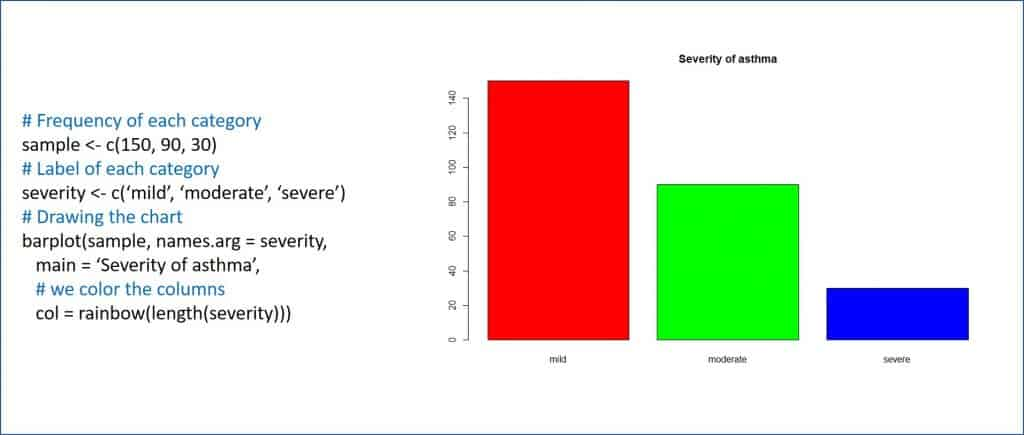With what has been seen so far, some will think that the title of this post is a bit misleading. Actually, the thing is not about columns and sectors, but about bars and pies. Also, who is the illustrious Italian? Well, here I do not fool anyone, because the character was both Italian and illustrious, and I am referring to Vilfredo Federico Pareto.

Pareto was an Italian who was born in the mid-19th century in Paris. This small contradiction is due to the fact that his father was then exiled in France for being one of the followers of Giuseppe Mazzini, who was then committed to Italian unification. Anyway, Pareto lived in Italy from he was 10 years old on, becoming an engineer with extensive mathematical and humanistic knowledge and who contributed decisively to the development of microeconomics. He spoke and wrote fluently in French, English, Italian, Latin and Greek, and became famous for a multitude of contributions such as the Pareto’s distribution, Pareto’s efficiency, Pareto’s index and Pareto’s principle. To represent the latter, he invented the Pareto’s diagram, which is what brings him here today among us.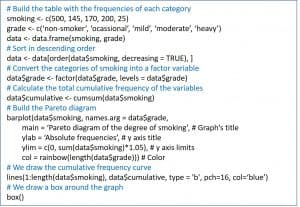Pareto chart (also known in economics as a closed curve or A-B-C distribution) organizes the data in descending order from left to right, represented by bars, thus assigning an order of priorities. In addition, the diagram incorporates a curved line that represents the cumulative frequency of the categories of the variable. This initially allowed the Pareto’s principle to be explained, which goes on to say that there are many minor problems compared to a few that are important, which was very useful for decision-making.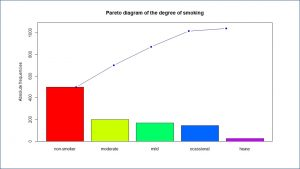As it is easy to understand, this prioritization makes the Pareto diagram especially useful for representing ordinal qualitative variables, surpassing the bar chart by giving information on the percentage accumulated by adding the categories of the distribution of the variable. The change in slope of this curve also informs us of the change in the concentration of data, which depends on the variability in which the subjects of the sample are divided between the different categories.

Unfortunately, R does not have a simple function to represent Pareto diagrams, but we can easily obtain it with the script that I attached in the third figure, obtaining the graph of the fourth.

And here we are going to leave it for today. Before saying goodbye, I want to warn you that you should not confuse the bars of the bar chart with those of the histogram since, although they can be similar from the graphic point of view, both represent very different things. In a bar chart only the values of the variables we have observed when doing the study are represented. However, the histogram goes much further since, in reality, it contains the frequency distribution of the variable, so it represents all possible values that exist within the intervals, although we have not observed any directly. It allows us to calculate the probability that any distribution value will be represented, which is of great importance if we want to make inference and estimate population values based on the results of our sample. But that is another story…

## Like a forgotten clock

I don’t like the end of summer. The days with bad weather begin, I wake up completely in the dark and in the evening it gets dark early and early. And, as if this were not bad enough, the cumbersome moment of change between summer and winter time is approaching.

In addition to the inconvenience of the change and the tedium of being two or three days remembering what time it is and what it could be if it had not been any change, we must proceed to adjust a lot of clocks manually. And, no matter how much you try to change them all, you always leave some with the old hour. It does not happen to you with the kitchen clock, at which you always look to know how fast you have to have breakfast, or with the one in the car, which stares at you every morning. But surely there are some that you do not change. Even, it has ever happened to me, that I realize it when the next time to change I see that I don’t need to do it because I left it unchanged in the previous time.

These forgotten clocks remind me a little of categorical or qualitative variables.

You will think that, once again, I forgot to take my pill this morning, but no. Everything has its reasoning. When we finish a study and we already have the results, the first thing we do is a description of them and then go on to do all kinds of contrasts, if applicable.

Well, qualitative variables are always belittled when we apply our knowledge of descriptive statistics. We usually limit ourselves to classifying them and making frequency tables with which to calculate some indices as their relative or accumulated frequency, to give some representative measure such as mode and little else. We use to work a little more with its graphic representation with bar or sector diagrams, pictograms and other similar inventions. And finally, we apply a little more effort when we relate two qualitative variables through a contingency table.

However, we forget their variability, something we would never do with a quantitative variable. The quantitative variables are like that kitchen wall clock that looks us straight in the eye every morning and does not allow us to leave it out of time. Therefore, we use these concepts we understand very well as the mean and variance or standard deviation. But that we do not know how to objectively measure the variability of qualitative or categorical variables, whether nominal or ordinal, does not mean that it does not exist a way to do it. For this purpose, several diversity indexes have been developed, which some authors distinguish as dispersion, variability and disparity indexes. Let’s see some of them, whose formulas you can see in the attached box, so you can enjoy the beauty of mathematical language.

The two best known indexes used to measure the variability or diversity are the Blau’s index (or of Hirschman- Herfindal’s) and the entropy index (or Teachman’s). Both have a very similar meaning and, in fact, are linearly correlated.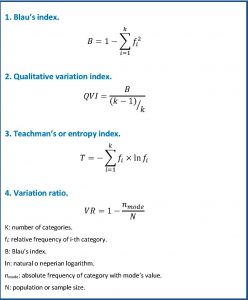Blau’s index quantifies the probability that two individuals chosen at random from a population are in different categories of a variable (provided that the population size is infinite or the sampling is performed with replacement). Its minimum value, zero, would indicate that all members are in the same category, so there would be no variety. The higher its value, the more dispersed among the different categories of the variable will be the components of the group. This maximum value is reached when the components are distributed equally among all categories (their relative frequencies are equal). Its maximum value would be (k-1) / k, which is a function of k (the number of categories of the qualitative variable) and not of the population size. This value tends to 1 as the number of categories increases (to put it more correctly, when k tends to infinity).

Let’s look at some examples to clarify it a bit. If you look at the Blau’s index formula, the value of the sum of the squares of the relative frequencies in a totally homogeneous population will be 1, so the index will be 0. There will only be one category with frequency 1 (100%) and the rest with zero frequency.

As we have said, although the subjects are distributed similarly in all categories, the index increases as the number of categories increases. For example, if there are four categories with a frequency of 0.25, the index will be 0.75 (1 – (4 x 0.252)). If there are five categories with a frequency of 0.2, the index will be 0.8 (1 – (5 x 0.22). And so on.

As a practical example, imagine a disease in which there is diversity from the genetic point of view. In a city A, 85% of patients has genotype 1 and 15% genotype 2. The Blau’s index values 1 – (0.85+ 0.152) = 0.255. In view of this result, we can say that, although it is not homogeneous, the degree of heterogeneity is not very high.

Now imagine a city B with 60% of genotype 1, 25% of genotype 2 and 15% of genotype 3. The Blau’s index will be 1 – (0.6x 0.252 x 0.152) = 0.555. Clearly, the degree of heterogeneity is greater among the patients of city B than among those of A. The smartest of you will tell me that that was already clear without calculating the index, but you have to take into account that I chose a very simple example for not giving my all calculating. In real-life, more complex studies, it is not usually so obvious and, in any case, it is always more objective to quantify the measure than to remain with our subjective impression.

This index could also be used to compare the diversity of two different variables (as long as it makes sense to do so) but, the fact that its maximum value depends on the number of categories of the variable, and not on the size of the sample or population, questions its usefulness to compare the diversity of variables with different number of categories. To avoid this problem, the Blau’s index can be normalized by dividing it by its maximum, thus obtaining the qualitative variation index. Its meaning is, of course, the same as that of the Blau’s index and its value ranges between 0 and 1. Thus, we can use either one if we compare the diversity of two variables with the same number of categories, but it will be more correct to use the qualitative variation index if the variables have a different number of categories.

The other index, somewhat less famous, is the Teachman’s index or entropy index , whose formula is also attached. Very briefly we will say that its minimum value, which is zero, indicates that there are no differences between the components in the variable of interest (the population is homogeneous). Its maximum value can be estimated as the negative value of the neperian logarithm of the inverse of the number of categories (- ln ( 1 / k)) and is reached when all categories have the same relative frequency (entropy reaches its maximum value). As you can see, very similar to Blau’s, which is much easier to calculate than Teachman’s.

To end this entry, the third index that I want to talk about today tells us, more than about the variability of the population, about the dispersion that its components have regarding the most frequent value. This can be measured by the variation ratio, which indicates the degree to which the observed values ​​do not coincide with that of mode, which is the most frequent category. As with the previous ones, I also show the formula in the attached box.

In order not to clash with the previous ones, its minimum value is also zero and is obtained when all cases coincide with the mode. The lower the value, the less the dispersion. The lower the absolute frequency of the mode, the closer it will be to 1, the value that indicates maximum dispersion. I think this index is very simple, so we are not going to devote more attention to it.

And we have reached the end of this post. I hope that from now on we will pay more attention to the descriptive analysis of the results of the qualitative variables. Of course, it would be necessary to complete it with an adequate graphic description using the well-known bar or sector diagrams (the pies) and others less known as the Pareto’s diagrams. But that is another story…

## Worshipped, but misunderstood

Statistics wears most of us who call ourselves “clinicians” out. The knowledge on the subject acquired during our formative years has long lived in the foggy world of oblivion. We vaguely remember terms such as probability distribution, hypothesis contrast, analysis of variance, regression … It is for this reason that we are always a bit apprehensive when we come to the methods section of scientific articles, in which all these techniques are detailed that, although they are known to us, we do not know with enough depth to correctly interpret their results.

Fortunately, Providence has given us a lifebelt: our beloved and worshipped p. Who has not felt lost with a cumbersome description of mathematical methods to finally breathe a sigh of relieve when finding the value of p? Especially if the p is small and has many zeros.

The problem with p is that, although it is unanimously worshipped, it is also mostly misunderstood. Its value is, very often, misinterpreted. And this is so because many of us harbor misconceptions about what the p-value really means.

Let’s try to clarify it.

Whenever we want to know something about a variable, the effect of an exposure, the comparison of two treatments, etc., we will face the ubiquity of random: it is everywhere and we can never get rid of it, although we can try to limit it and, of course, try to measure its effect.

Let’s give an example to understand it better. Suppose we are doing a clinical trial to compare the effect of two diets, A and B, on weight gain in two groups of participants. Simplifying, the trial will have one of three outcomes: those of diet A gain more weight, those of diet B gain more weight, both groups gain equal weight (there could even be a fourth: both groups lose weight). In any case, we will always obtain a different result, just by chance (even if the two diets are the same).

Imagine that those in diet A put on 2 kg and those in diet B, 3 kg. Is it more fattening the effect of diet B or is the difference due to chance (chosen samples, biological variability, inaccuracy of measurements, etc.)? This is where our hypothesis contrast comes in.

When we are going to do the test, we start from the hypothesis of equality, of no difference in effect (the two diets induce the same increment of weight). This is what we call the null hypothesis (H0) that, I repeat it to keep it clear, we assume that it is the real one. If the variable we are measuring follows a known probability distribution (normal, chi-square, Student’s t, etc.), we can calculate the probability of presenting each of the values of the distribution. In other words, we can calculate the probability of obtaining a result as different from equality as we have obtained, always under the assumption of H0.

That is the p-value: the probability that the difference in the result observed is due to chance. By agreement, if that probability is less than 5% (0.05) it will seem unlikely that the difference is due to chance and we will reject H0, the equality hypothesis, accepting the alternative hypothesis (Ha) that, in this example, will say that one diet better than the other. On the other hand, if the probability is greater than 5%, we will not feel confident enough to affirm that the difference is not due to chance, so we DO NOT reject H0 and we keep with the hypothesis of equal effects: the two diets are similar.

Keep in mind that we always move in the realm of probability. If p is less than 0.05 (statistically significant), we will reject H0, but always with a probability of committing a type 1 error: take for granted an effect that, in reality, does not exist (a false positive). On the other hand, if p is greater than 0.05, we keep with H0 and we say that there is no difference in effect, but always with a probability of committing a type 2 error: not detecting an effect that actually exists (false negative).

We can see, therefore, that the value of p is somewhat simple from the conceptual point of view. However, there are a number of common errors about what p-value represents or does not represent. Let’s try to clarify them.

It is false that a p-value less than 0.05 means that the null hypothesis is false and a p-value greater than 0.05 that the null hypothesis is true. As we have already mentioned, the approach is always probabilistic. The p <0.05 only means that, by agreement, it is unlikely that H0 is true, so we reject it, although always with a small probability of being wrong. On the other hand, if p> 0.05, it is also not guaranteed that H0 is true, since there may be a real effect that the study does not have sufficient power to detect.

At this point we must emphasize one fact: the null hypothesis is only falsifiable. This means that we can only reject it (with which we keep with Ha, with a probability of error), but we can never affirm that it is true. If p> 0.05 we cannot reject it, so we will remain in the initial assumption of equality of effect, which we cannot demonstrate in a positive way.

It is false that p-value is related to the reliability of the study. We can think that the conclusions of the study will be more reliable the lower the value of p, but it is not true either. Actually, the p-value is the probability of obtaining a similar value by chance if we repeat the experiment in the same conditions and it not only depends on whether the effect we want to demonstrate exists or not. There are other factors that can influence the magnitude of the p-value: the sample size, the effect size, the variance of the measured variable, the probability distribution used, etc.

It is false that p-value indicates the relevance of the result. As we have already repeated several times, p-value is only the probability that the difference observed is due to chance. A statistically significant difference does not necessarily have to be clinically relevant. Clinical relevance is established by the researcher and it is possible to find results with a very small p that are not relevant from the clinical point of view and vice versa, insignificant values that are clinically relevant.

It is false that p-value represents the probability that the null hypothesis is true. This belief is why, sometimes, we look for the exact value of p and do not settle for knowing only if it is greater or less than 0.05. The fault of this error of concept is a misinterpretation of conditional probability. We are interested in knowing what is the probability that H0 is true once we have obtained some results with our test. Mathematically expressed, we want to know P (H0 | results). However, the value of p gives us the probability of obtaining our results under the assumption that the null hypothesis is true, that is, P (results | H0).

Therefore, if we interpret that the probability that H0 is true in view of our results (P (H0 | results)) is equal to the value of p (P (results | H0)) we will be falling into an inverse fallacy or transposition of conditionals fallacy.

In fact, the probability that H0 is true does not depend only on the results of the study, but is also influenced by the previous probability that was estimated before the study, which is a measure of the subjective belief that reflects its plausibility, generally based on previous studies and knowledge. Let’s think we want to contrast an effect that we believe is very unlikely to be true. We will value with caution a p-value <0.05, even being significant. On the contrary, if we are convinced that the effect exists, will be settle for with little demands of p-value.

In summary, to calculate the probability that the effect is real we must calibrate the p-value with the value of the baseline probability of H0, which will be assigned by the researcher or by previously available data. There are mathematical methods to calculate this probability based on its baseline probability and the p-value, but the simplest way is to use a graphical tool, the Held’s nomogram, which you can see in the figure.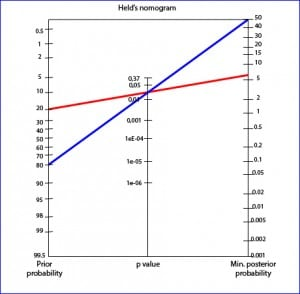To use the Held’s nomogram we just have to draw a line from the previous H0 probability that we consider to the p-value and extend it to see what posterior probability value we reach. As an example, we have represented a study with a p-value = 0.03 in which we believe that the probability of H0 is 20% (we believe there is 80% that the effect is real). If we extend the line it will tell us that the minimum probability of H0 is 6%: there is a 94% probability that the effect is real. On the other hand, think of another study with the same p-value but in which we think that the probability of the effect is lower, for example, of 20% (the probability of H0 is 80%). For the same value of p, the minimum posterior probability of H0 is 50%, then there is 50% that the effect is real. As we can see, the posterior probability changes according to the previous probability.

And here we will end for today. We have seen how p-value only gives us an idea of the role that chance may have had in our results and that, in addition, may depend on other factors, perhaps the most important the sample size. The conclusion is that, in many cases, the p-value is a parameter that allows to assess in a very limited way the relevance of the results of a study. To do it better, it is preferable to resort to the use of confidence intervals, which will allow us to assess clinical relevance and statistical significance. But that is another story…

## The cheaters detector

When we think about inventions and inventors, the name of Thomas Alva Edison, known among his friends as the Wizard of Menlo Park, comes to most of us. This gentleman created more than a thousand inventions, some of which can be said to have changed the world. Among them we can name the incandescent bulb, the phonograph, the kinetoscope, the polygraph, the quadruplex telegraph, etc., etc., etc. But perhaps its great merit is not to have invented all these things, but to apply methods of chain production and teamwork to the research process, favoring the dissemination of their inventions and the creation of the first industrial research laboratory.

But in spite of all his genius and excellence, Edison failed to go on to invent something that would have been as useful as the light bulb: a cheaters detector. The explanation for this pitfall is twofold: he lived between the nineteenth and twentieth centuries and did not read articles about medicine. If he had lived in our time and had to read medical literature, I have no doubt that the Wizard of Menlo Park would have realized the usefulness of this invention and would have pull his socks up.

And it is not that I am especially negative today, the problem is that, as Altman said more than 15 years ago, the material sent to medical journals is defective from the methodological point of view in a very high percentage of cases. It’s sad, but the most appropriate place to store many of the published studies is the rubbish can.

In most cases the cause is probably the ignorance of those who write. “We are clinicians”, we say, so we leave aside the methodological aspects, of which we have a knowledge, in general, quite deficient. To fix it, journal editors send our studies to other colleagues, who are more or less like us. “We are clinicians”, they say, so all our mistakes go unnoticed to them.

Although this is, in itself, serious, it can be remedied by studying. But it is an even more serious fact that, sometimes, these errors can be intentional with the aim of inducing the reader to reach a certain conclusion after reading the article. The remedy for this problem is to make a critical appraisal of the study, paying attention to its internal validity. In this sense, perhaps the most difficult aspect to assess for the clinician without methodological training is that related to the statistics used to analyze the results of the study. It is in this, undoubtedly, that most can be taken advantage of our ignorance using methods that provide more striking results, instead of the right methods.

As I know that you are not going to be willing to do a master’s degree in biostatistics, waiting for someone to invent the cheaters detector, we are going to give a series of clues so that non-expert readers can suspect the existence of these cheats.

The first may seem obvious, but it is not: has a statistical method been used? Although it is exceptionally rare, there may be authors who do not consider using any. I remember a medical congress that I could attend in which the values of a variable were exposed throughout the study that, first, went up and then went down, which allowed the speaker to conclude that the result was not “on the blink”. As it is logical and evident, any comparison must be made with the proper hypotheses contrast and the level of significance and the statistical test used have to be specified. Otherwise, the conclusions will lack any validity.

A key aspect of any study, especially those with an intervention, is the previous calculation of the necessary sample size. The investigator must define the clinically relevant effect that he wants to be able to detect with his study and then calculate what sample size will provide the study with enough power to prove it. The sample of a study is not large or small, but sufficient or insufficient. If the sample is not sufficient, an existing effect may not be detected due to lack of power (type 2 error). On the other hand, a larger sample than necessary may show an effect that is not relevant from the clinical point of view as statistically significant. Here are two very common cheats. First, the study that does not reach significance and its authors say it is due to lack of power (insufficient sample size), but do not make any effort to calculate the power, which can always be done a posteriori. In that case, we can calculate it using statistical programs or any of the calculators available on the internet, such as GRANMO. Second, the sample size is increased until the difference observed is significant, finding the desired p <0.05. This case is simpler: we only have to assess whether the effect found is relevant from the clinical point of view. I advise you to practice and compare the necessary sample sizes of the studies with those defined by the authors. Maybe you’ll have some surprise.

Once the participants have been selected, a fundamental aspect is that of the homogeneity of the basal groups. This is especially important in the case of clinical trials: if we want to be sure that the observed difference in effect between the two groups is due to the intervention, the two groups should be the same in everything, except in the intervention.

For this we will look at the classic table I of the trial publication. Here we have to say that, if we have distributed the participants at random between the two groups, any difference between them will be due, one way or another, to random. Do not be fooled by the p, remember that the sample size is calculated for the clinically relevant magnitude of the main variable, not for the baseline characteristics of the two groups. If you see any difference and it seems clinically relevant, it will be necessary to verify that the authors have taken into account their influence on the results of the study and have made the appropriate adjustment during the analysis phase.

The next point is that of randomization. This is a fundamental part of any clinical trial, so it must be clearly defined how it was done. Here I have to tell you that chance is capricious and has many vices, but rarely produces groups of equal size. Think for a moment if you flip a coin 100 times. Although the probability of getting heads in each throw is 50%, it will be very rare that by throwing 100 times you will get exactly 50 heads. The greater the number of participants, the more suspicious it should seem to us that the two groups are equal. But beware, this only applies to simple randomization. There are methods of randomization in which groups can be more balanced.

Another hot spot is the misuse that can sometimes be made with qualitative variables. Although qualitative variables can be coded with numbers, be very careful with doing arithmetic operations with them. Probably it will not make any sense. Another cheat that we can find has to do with the fact of categorizing a continuous variable. Passing a continuous variable to a qualitative one usually leads to loss of information, so it must have a clear clinical meaning. Otherwise, we can suspect that the reason is the search for a p value less than 0.05, always easier to achieve with the qualitative variable.

Going into the analysis of the data, we must check that the authors have followed the a priori designed protocol of the study. Always be wary of post hoc studies that were not planned from the beginning. If we look for enough, we will always find a group that behaves as we want. As it is said, if you torture the data long enough, it will confess to anything.

Another unacceptable behavior is to finish the study ahead of time for good results. Once again, if the duration of the follow-up has been established during the design phase as the best time to detect the effect, this must be respected. Any violation of the protocol must be more than justified. Logically, it is ethical to finish the study ahead of time due to security reasons, but it will be necessary to take into account how this fact affects the evaluation of the results.

Before performing the analysis of the results, the authors of any study have to debug their data, reviewing the quality and integrity of the values collected. In this sense, one of the aspects to pay attention to is the management of outliers. These are the values that are far from the central values of the distribution. In many occasions they can be due to errors in the calculation, measurement or transcription of the value of the variable, but they can also be real values that are due to the special idiosyncrasy of the variable. The problem is that there is a tendency to eliminate them from the analysis even when there is no certainty that they are due to an error. The correct thing to do is to take them into account when doing the analysis and use, if necessary, robust statistical methods that allow these deviations to be adjusted.

Finally, the aspect that can be more strenuous to those not very expert in statistics is knowing if the correct statistical method has been used. A frequent error is the use of parametric tests without previously checking if the necessary requirements are met. This can be done by ignorance or to obtain statistical significance, since parametric tests are less demanding in this regard. To understand each other, the p-value will be smaller than if we use the equivalent non-parametric test.

Also, with certain frequency, other requirements needed to be able to apply a certain contrast test are ignored. As an example, in order to perform a Student’s t test or an ANOVA, homoscedasticity (a very ugly word that means that the variances are equal) must be checked, and that check is overlooked in many studies. The same happens with regression models that, frequently, are not accompanied by the mandatory diagnosis of the model that allows and justify its use.

Another issue in which there may be cheating is that of multiple comparisons. For example, when the ANOVA reaches significant, the meaning is that there are at least two means that are different, but we do not know which, so we start comparing them two by two. The problem is that when we make repeated comparisons the probability of type I error increases, that is, the probability of finding significant differences only by chance. This may allow finding, if only by chance, a p <0.05, what improves the appearance of the study (especially if you spent a lot of time and / or money doing it). In these cases, the authors must use some of the available corrections (such as Bonferroni’s, one of the simplest) so that the global alpha remains below 0.05. The price to pay is simple: the p-value has to be much smaller to be significant. When we see multiple comparisons without a correction, it will only have two explanations: the ignorance of the one who made the analysis or the attempt to find a statistical significance that, probably, would not support the decrease in p-value that the correction would entail.

Another frequent victim of misuse of statistics is the Pearson’s correlation coefficient, which is used for almost everything. The correlation, as such, tells us if two variables are related, but does not tell us anything about the causality of one variable for the production of the other. Another misuse is to use the correlation coefficient to compare the results obtained by two observers, when probably what should be used in this case is the intraclass correlation coefficient (for continuous variables) or the kappa index (for dichotomous qualitative variables). Finally, it is also incorrect to compare two measurement methods (for example, capillary and venous glycaemia) by correlation or linear regression. For these cases the correct thing would be to use the Passing-Bablok’s regression.

Another situation in which a paranoid mind like mine would suspect is one in which the statistical method employed is not known by the smartest people in the place. Whenever there is a better known (and often simpler) way to do the analysis, we must ask ourselves why they have used such a weird method. In these cases, we will require the authors to justify their choice and provide a reference where we can review the method. In statistics, you have to try to choose the right technique for each occasion and not the one that gives us the most appealing result.

In any of the previous contrast tests, the authors usually use a level of significance for p <0.05, as usual, but the contrast can be done with one or two tails. When we do a trial to try a new drug, what we expect is that it works better than the placebo or the drug with which we are comparing it. However, two other situations can occur that we cannot disdain: that it works the same or, even, that it works worse. A bilateral contrast (with two tails) does not assume the direction of the effect, since it calculates the probability of obtaining a difference equal to or greater than that observed, in both directions. If the researcher is very sure of the direction of the effect, he can make a unilateral contrast (with one tail), measuring the probability of the result in the direction considered. The problem is when he does it for another reason: the p-value of a bilateral contrast is twice as large as that of the unilateral contrast, so it will be easier to achieve statistical significance with the unilateral contrast. The wrong thing is to do the unilateral contrast for that reason. The correct thing, unless there are well-justified reasons, is to make a bilateral contrast.

To go finishing this tricky post, we will say a few words about the use of appropriate measures to present the results. There are many ways to make up the truth without getting to lie and, although basically all say the same, the appearance can be very different depending on how we say it. The most typical example is to use relative risk measures instead of absolute and impact measures. Whenever we see a clinical trial, we must demand that authors provide the absolute risk reduction and the number needed to treat (NNT). The relative risk reduction gives a greater number than the absolute, so it will seem that the impact is greater. Given that the absolute measures are easier to calculate and are obtained from the same data as the relative ones, we should be suspicious if the authors do not offer them to us: perhaps the effect is not as important as they are trying to make us see.

Another example is the use of odds ratio versus risk ratio (when both can be calculated). The odds ratio tends to magnify the association between the variables, so its unjustified use can also make us to be suspicious. If you can, calculate the risk ratio and compare the two measures.

Likewise, we will suspect of studies of diagnostic tests that do not provide us with the likelihood ratios and are limited to sensitivity, specificity and predictive values. Predictive values can be high if the prevalence of the disease in the study population is high, but it would not be applicable to populations with a lower proportion of patients. This is avoided with the use of likelihood ratios. We should always ask ourselves the reason that the authors may have had to obviate the most valid parameter to calibrate the power of a diagnostic test.

And finally, be very careful with the graphics representations of results: here the possibilities of making up the truth are only limited by our imagination. You have to look at the units used and try to extract the information from the graph beyond what it might seem to represent at first glance.

And here we leave the topic for today. We have not spoken in detail about another of the most misunderstood and manipulated entities, which is none other than our p. Many meanings are attributed to p, usually erroneously, as the probability that the null hypothesis is true, probability that has its specific method to make an estimate. But that is another story…